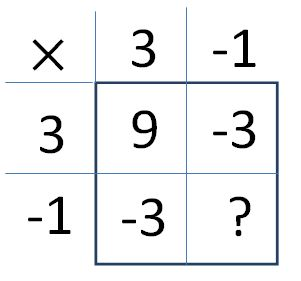# Why does a negative multiplied by a negative equal a positive?

20 November 2012When I was at school I remember my first unit of work on negative numbers. In the second lesson, our teacher wrote the note above on the chalkboard and asked us to memorise it:

“WHAT???” I thought. “This is just Great! (sarcastic tone) I don’t have a clue what’s going on now!”

I had no idea what it meant but I just wrote it down and hoped it would come to me later on in the lesson. Fortunately, I figured out a few minutes later that it was a set of rules to help with multiplying negative and positive numbers together. Once I’d deciphered the note, I was then puzzled by the second line:

“So, a negative number multiplied by a negative number is a positive number. How is that possible?”

I didn’t often question things in maths at School. In fact, I never questioned anything out loud. I’m not entirely sure why but I think the simple answer would be that we often accept the reality with which we’re presented. I’d always been taught that mathematics was about memorising, not understanding, and that consequently was my attitude towards the subject. To be completely honest, whilst I believe it did me no good at all, I was quite happy memorising formulas or concepts and then doing lots of practice questions until it stayed in there long enough to remember for the test.

Anyway, enough about my experiences – let’s get onto the topic of the post: why does a negative multiplied by a negative equal a positive?

To answer this, consider the simple multiplication, 2 x 2

Because I’m interested in finding out about negative numbers, I’m going to construct a situation in which I can start to investigate. Thus, instead of writing the multiplication as I did above, I’m going to change the format slightly to this:

(3 – 1) x (3 – 1)

Now we’re going to do a double bracket expansion on this. I like using the grid method but any method will do (FOIL, smiley face, etc.).Multiplying 3 and -1 is intuitively -3 so the only one I’m not sure of now in the grid is -1 x -1?

Now, we already know that the answer to 2 x 2 = 4

i.e.                          (3 – 1)(3 – 1) = 4

Since we’ve just expanded these brackets out, we therefore know that:

9 + -3 + -3 + ? = 4

Therefore,                                   3 + ? = 4

And in conclusion,  ? must be equal to 1 for this equation to be true which means that -1 x -1 = 1. Logically, it’s the only answer that makes sense. If -1 x -1 = -1, then 3 + -1 = 4, or put another way,      2 =  4 which we know to be incorrect.

We could have done it all with algebra but I personally prefer seeing a numerical example. I hope that’s helped a little with understanding why a negative multiplied by a negative is a positive. I guess the question now is whether I would have understood this technique at 11 years old? Would it just have confused me more? Interesting…

Dan Pearcy

(danpearcymaths.wordpress.com)

(Unfortunately I have no recollection of how I came to know about this method. It seemed to just come out of the ether as many things do nowadays. If you know who was the first person in history to explain it like this then please get in touch and they shall be rightly credited in this post)